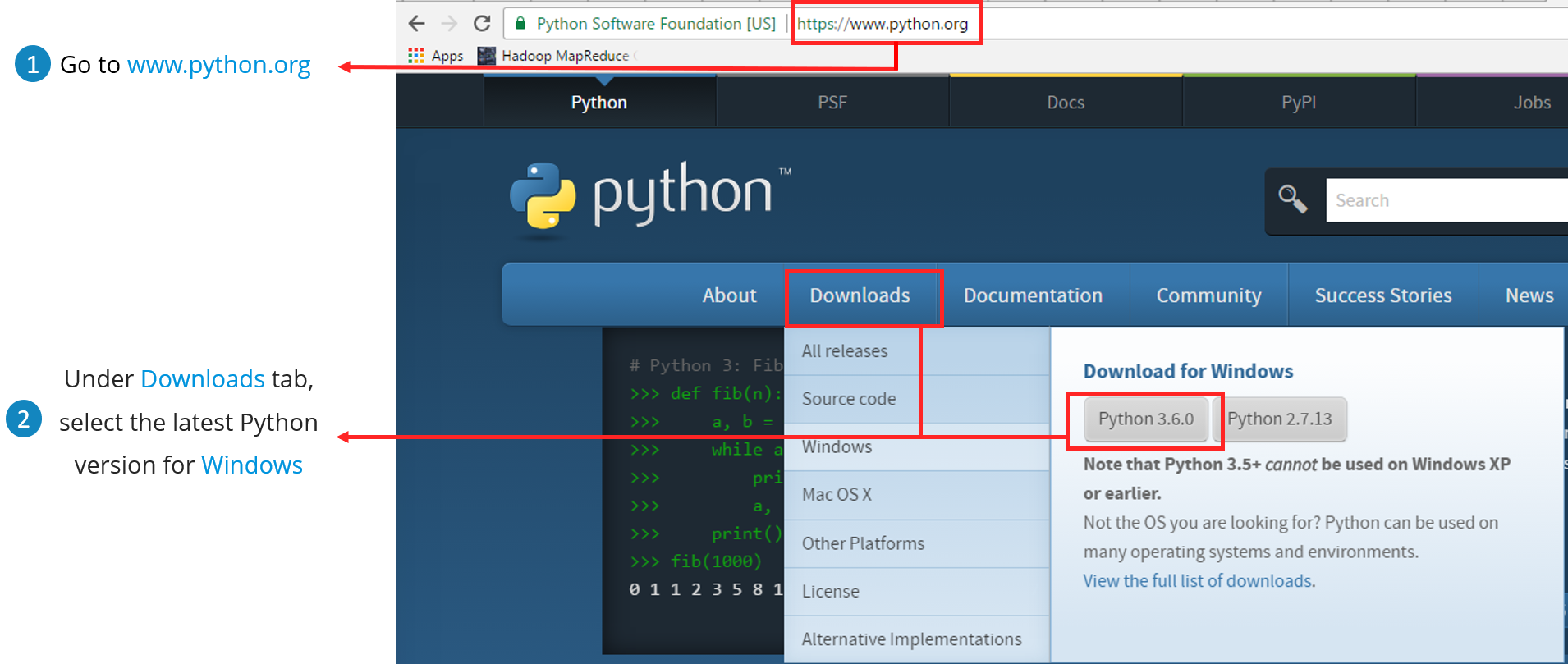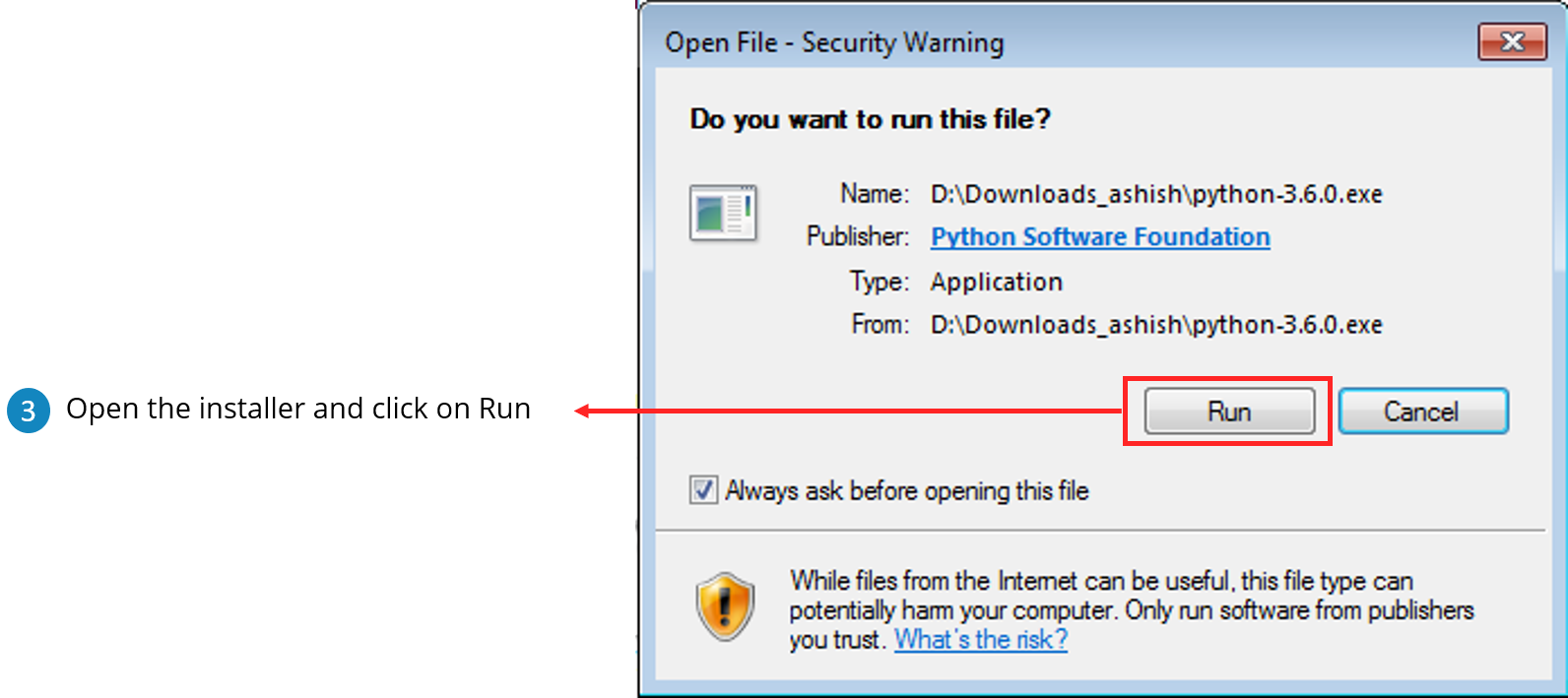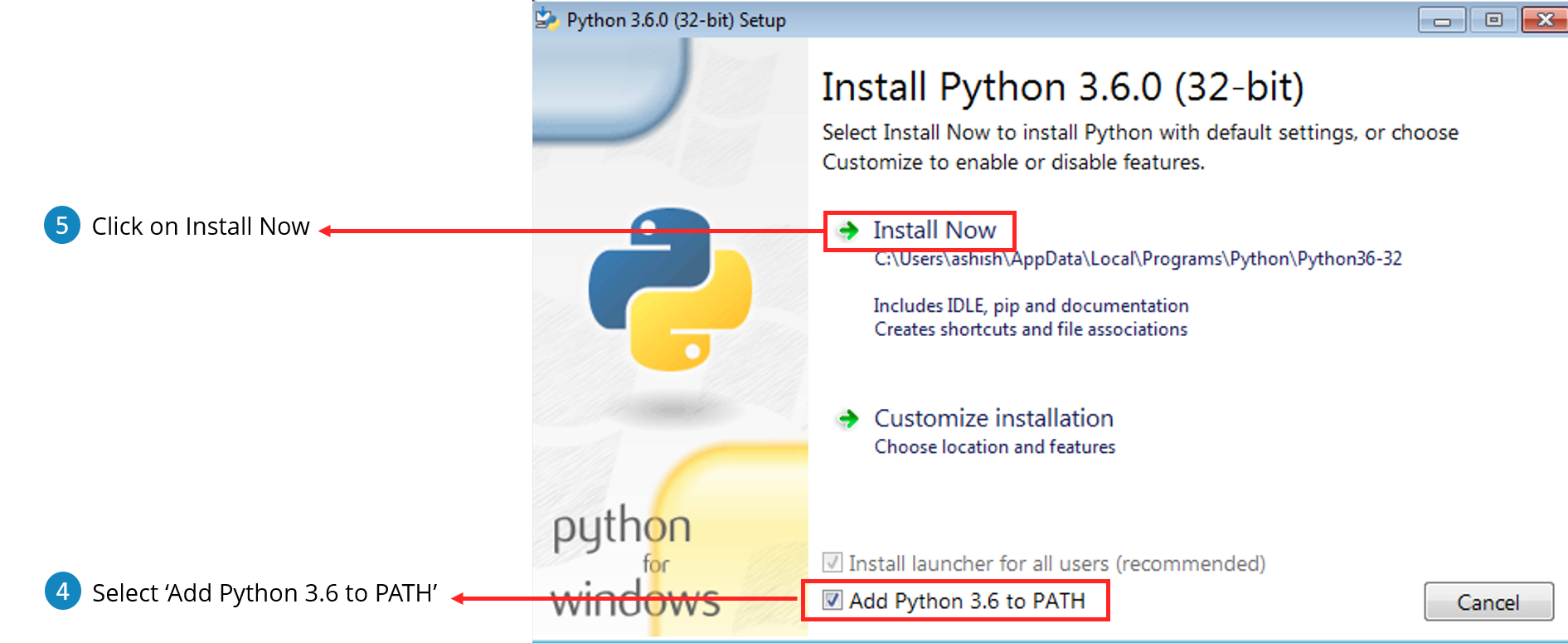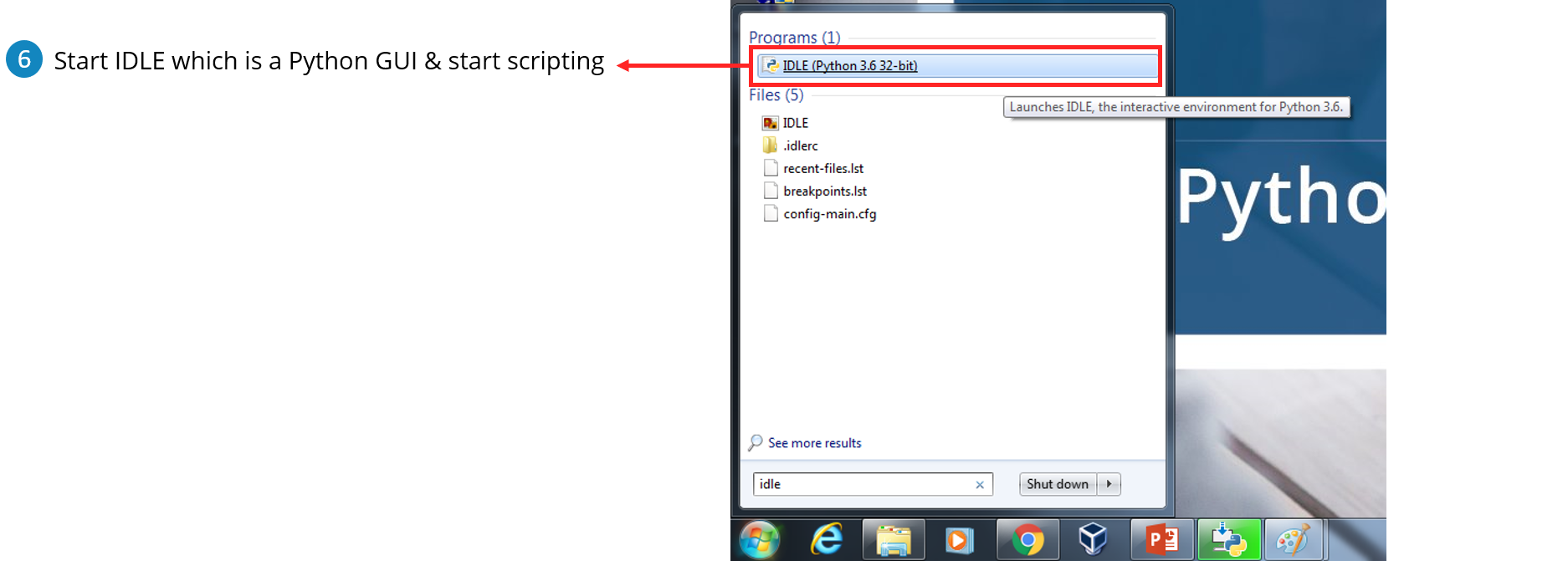# A Complete Guide to Learn Python ProgrammingIn this Python Tutorial will help you learn Python programming basics - Variables, Data types, Operators, Conditional Statements, Loops, Operations, Functions and more

Python is a high-level, object-oriented, interpreted programming language, which has garnered worldwide attention. Stack Overflow found out that 38.8% of its users mainly use Python for their projects. According to the website’s survey, Python’s popularity surpassed that of C# in 2018 – just like it surpassed PHP in 2017. On the GitHub platform, Python surpassed Java as the second-most used programming language, with 40% more pull requests opened in 2017 than in 2016. In this Python Tutorial, I will be discussing the following topics:

1. What is Python?
2. Python Features
3. Python Applications
4. Python and PyCharm Installation
5. Python IDE
6. Python Code Basics
1. Python Variables
2. Python Data Types
1. Lists
2. Tuples
3. Sets
4. Dictionary
5. Strings
6. Numeric
3. Python Operators
4. Python Conditional Statements
1. If Statement
2. Elif Statement
3. Else Statement
5. Python Loops
1. While Loop
2. For Loop
3. Nested Loop
6. I/O Operations
7. Python Functions

## What is Python?

As I have mentioned Python is an open-source object-oriented programming language. It first appeared in 1991 and has become extremely popular among data scientists. StackOverflow calls it as the fastest growing programming language.But, what I mean by interpreted language, let’s understand what is an interpreter first.

## Python Interpreter:

An interpreter is a computer program that directly executes, i.e. performs, instructions written in a programming or scripting language, without requiring them previously to have been compiled into a machine language program. So unlike Java, Python uses an interpreter.

Let us now install Python.

## Python Installation:

I will be installing Python in Windows 10 OS. You can try installing Python in Linux, Mac etc. If you face any issue mention it in the comments section.

Following are the steps to install Python2. Select the Operating System and also the version of Python. I am downloading 3.6.0 in my windows machine. Open the installer and click on “Run”.3. Click on “Install Now” and check on “Add Python 3.6 to PATH”.4. Start IDLE which is a Python GUI and start scripting.I don’t prefer using IDLE for coding in Python, instead, I will download PyCharm which is an IDE (Integrated Development Environment). It will only be fair if I explain to you what is an IDE before I proceed with this Python tutorial blog.

## Python IDE (Integrated Development Environment):

IDE typically provides code editor, compiler/ interpreter and debugger in one GUI (Graphical User Interface). It encapsulates the entire process of code creation, compilation and testing which increases the productivity of developers.

A developer working with an IDE starts with a model, which the IDE translates into suitable code. The IDE then debugs and tests the model-driven code, with a high level of automation. Once the build is successful and properly tested, it can be deployed for further testing through the IDE or other tools outside of the IDE.

I am going to use PyCharm, you can use any other IDE that you want.

### Installing PyCharm (Python IDE):Here, the community version is free, but for the professional version, you need to buy the license. I will be working on the PyCharm community version.

Now, let us have a look at why one should even consider Python as a preferred or first programming language.

## Why Learn Python?

Python’s syntax is very easy to understand. The lines of code required for a task is less compared to other languages. Let me give you an example – If I have to print “Welcome To Mmorioh!” all I have to type:

``````print (“Welcome To Morioh!”)
``````

Let’s look at some cool features of Python:

1. Simple and easy to learn
2. Free and Open Source
3. Portable
5. Extensible

If you are wondering where you can use Python (Python Application), let me tell you that is where Python stands out.

## Python Applications:

1. Artificial Intelligence
2. Desktop Application
3. Automation
4. Web Development
5. Data Wrangling, Exploration And Visualization

Let us now start coding in Python, as I have mentioned above I will be using PyCharm.

## Variables in Python:

Variables are nothing but reserved memory locations to store values. This means that when you create a variable you reserve some space in memory.Fig: The figure above shows three variables A, B and C

In Python you don’t need to declare variables before using it, unlike other languages like Java, C etc.

### Assigning values to a variable:

Python variables do not need explicit declaration to reserve memory space. The declaration happens automatically when you assign a value to a variable. The equal sign (=) is used to assign values to variables. Consider the below example:

``````S = 10
print(S)
``````

This will assign value ‘10’ to the variable ‘S’ and will print it. Try it yourself.

Now in this Python Tutorial, we’ll understand Data types.

## Data Types in Python:

Python supports various data types, these data types defines the operations possible on the variables and the storage method. Below is the list of standard data types available in Python:Let’s discuss each of these in detail. In this Python tutorial, we’ll start with ‘Numeric’ data type.

### Numeric:

Just as expected Numeric data types store numeric values. They are immutable data types, this means that you cannot change it’s value. Python supports three different Numeric data types:

Integer type: It holds all the integer values i.e. all the positive and negative whole numbers, example – 10.

Float type: It holds the real numbers and are represented by decimal and sometimes even scientific notations with E or e indicating the power of 10 (2.5e2 = 2.5 x 102 = 250), example – 10.24.

Complex type: These are of the form a + bj, where a and b are floats and J represents the square root of -1 (which is an imaginary number), example – 10+6j.

Now you can even perform type conversion. For example, you can convert the integer value to a float value and vice-versa. Consider the example below:

``````A = 10
# Convert it into float type
B = float(A)
print(B)
``````

The code above will convert an integer value to a float type. Similarly you can convert a float value to integer type:

``````A = 10.76
# Convert it into float type
B = int(A)
print(B)
``````

Now let’s understand what exactly are lists in this Python Tutorial.

### List

• You can consider the Lists as Arrays in C, but in List you can store elements of different types, but in Array all the elements should of the same type.
• List is the most versatile datatype available in Python which can be written as a list of comma-separated values (items) between square brackets. Consider the example below
``````Subjects = ['Physics', 'Chemistry', 'Maths', 2]
print(Subjects)
``````

Notice that the Subjects List contains both words as well as numbers. Now, let’s perform some operations on our Subjects List.

Let’s look at few operations that you can perform with Lists:Next in Python Tutorial, let’s focus on Tuples.

### Tuples:

A Tuple is a sequence of immutable Python objects. Tuples are sequences, just like Lists. The differences between tuples and lists are:

• Tuples cannot be changed unlike lists
• Tuples use parentheses, whereas lists use square brackets. Consider the example below:
``````Chelsea = ('Hazard', 'Lampard', 'Terry')
``````

Now you must be thinking why Tuples when we have Lists?

So the simple answer would be, Tuples are faster than Lists. If you’re defining a constant set of values which you just want to iterate, then use Tuple instead of a List.

Guys, all Tuple operations are similar to Lists, but you cannot update, delete or add an element to a Tuple.

Now, stop being lazy and don’t expect me to show all those operations, try it yourself.

Next in Python Tutorial, let’s understand Strings.

### Strings:

Strings are amongst the most popular data types in Python. We can create them simply by enclosing characters in quotes. Python treats single and double quotes in exactly the same fashion. Consider the example below:

``````S = "Welcome To Morioh!"
D = 'Morioh!'
``````

Let’s look at few operations that you can perform with Strings.I hope you have enjoyed the read till now. Next up, in this Python tutorial we will focus on Set.

### Set:

• A Set is an unordered collection of items. Every element is unique.
• A Set is created by placing all the items (elements) inside curly braces {}, separated by comma. Consider the example below:
``````Set_1 = {1, 2, 3}
``````

In Sets, every element has to be unique. Try printing the below code:

``````Set_2 = {1, 2, 3, 3}
``````

Here 3 is repeated twice, but it will print it only once.

Let’s look at some Set operations:

#### Union:

Union of A and B is a set of all the elements from both sets. Union is performed using | operator. Consider the below example:

``````A = {1, 2, 3, 4}
B = {3, 4, 5, 6}
print ( A | B)
``````

Output = {1, 2, 3, 4, 5, 6}

#### Intersection:Intersection of A and B is a set of elements that are common in both sets. Intersection is performed using & operator. Consider the example below:

``````A = {1, 2, 3, 4}
B = {3, 4, 5, 6}
print ( A & B )
``````

Output = {3, 4}

#### Difference:Difference of A and B (A – B) is a set of elements that are only in A but not in B. Similarly, B – A is a set of element in B but not in A. Consider the example below:

``````A = {1, 2, 3, 4, 5}
B = {4, 5, 6, 7, 8}
print(A - B)
``````

Output = {1, 2, 3}

#### Symmetric Difference:Symmetric Difference of A and B is a set of elements in both A and B except those that are common in both. Symmetric difference is performed using ^ operator. Consider the example below:

``````A = {1, 2, 3, 4, 5}
B = {4, 5, 6, 7, 8}
print(A ^ B)
``````

Output = {1, 2, 3, 6, 7, 8}

Next in Python Tutorial, it’s the time to focus on the last Data type i.e. Dictionary

### Dictionary:

Now let me explain you Dictionaries with an example.

I am guessing you guys know about Adhaar Card. For those of you who don’t know what it is, it is nothing but a unique ID which has been given to all Indian citizen. So for every Adhaar number, there is a name and few other details attached.

Now you can consider the Adhaar number as a ‘Key’ and the person’s detail as the ‘Value’ attached to that Key.

Dictionaries contains these ‘Key Value’ pairs enclosed within curly braces and Keys and values are separated with ‘:’. Consider the below example:

``````Dict = {'Name' : 'Saurabh', 'Age' : 23}
``````

You know the drill, now comes various Dictionary operations.

• Access elements from a dictionary:
``````Dict = {'Name' : 'Saurabh', 'Age' : 23}
print(Dict['Name'])
``````

Output = Saurabh

• Changing elements in a Dictionary:
``````Dict = {'Name' : 'Saurabh', 'Age' : 23}
Dict['Age'] = 32
``````

Output = {‘Name’ = ‘Saurabh’, ‘Age’ = 32, ‘Address’ = ‘Starc Tower’}

Next in Python Tutorial, let’s understand the various Operators in Python.

## Operators in Python:

Operators are the constructs which can manipulate the values of the operands. Consider the expression 2 + 3 = 5, here 2 and 3 are operands and + is called operator.

Python supports the following types of Operators:Let’s focus on each of these Operators one by one.

### Arithmetic Operators:

These Operators are used to perform mathematical operations like addition, subtraction etc. Assume that A = 10 and B = 20 for the below table.Consider the example below:

``````a = 21
b = 10
c = 0

c = a + b
print ( c )

c = a - b
print ( c )

c = a * b
print ( c )

c = a / b
print ( c )

c = a % b
print ( c )
a = 2
b = 3
c = a**b
print ( c )
``````

Output = 31, 11, 210, 2.1, 1, 8

Now let’s see comparison Operators.

#### Comparison Operators:

These Operators compare the values on either sides of them and decide the relation among them. Assume A = 10 and B = 20.Consider the example below:

``````a = 21
b = 10
c = 0

if ( a == b ):
print ("a is equal to b")
else:
print ("a is not equal to b")

if ( a != b ):
print ("a is not equal to b")
else:
print ("a is equal to b")

if ( a < b ): print ("a is less than b")
else: print ("a is not less than b")

if ( a > b ):
print ("a is greater than b")
else:
print ("a is not greater than b")

a = 5
b = 20
if ( a <= b ): print ("a is either less than or equal to b")
else: print ("a is neither less than nor equal to b")

if ( a => b ):
print ("a is either greater than  or equal to b")
else:
print ("a is neither greater than  nor equal to b")
``````
``````Output = a is not equal to b
a is not equal to b
a is not less than b
a is greater than b
a is either less than or equal to b
b is either greater than or equal to b
``````

Now in the above example, I have used conditional statements (if, else). It basically means if the condition is true then execute the print statement, if not then execute the print statement inside else. We will understand these statements later in this Python Tutorial blog.

### Assignment Operators:

An Assignment Operator is the operator used to assign a new value to a variable. Assume A = 10 and B = 20 for the below table.Consider the example below:

``````a = 21
b = 10
c = 0

c = a + b
print ( c )

c += a
print ( c )

c *= a
print ( c )

c /= a
print ( c )

c  = 2
c %= a
print ( c )

c **= a
print ( c )
``````

Output = 31, 52, 1092, 52.0, 2, 2097152, 99864

### Bitwise Operators:

These operations directly manipulate bits. In all computers, numbers are represented with bits, a series of zeros and ones. In fact, pretty much everything in a computer is represented by bits. Consider the example shown below:Following are the Bitwise Operators supported by Python:Consider the example below:

``````a = 58        # 111010
b = 13        # 1101
c = 0

c = a & b
print ( c )   # 8 = 1000

c = a | b
print ( c )   # 63 = 111111

c = a ^ b
print ( c )   # 55 = 110111

c = a >> 2
print ( c )   # 232 = 11101000

c = a << 2
print ( c )   # 14 = 1110
``````

Output = 8,63,55,232,14

Next up, in this Python Tutorial we will focus on Logical Operators.

### Logical Operators:The following are the Logical Operators present in Python:Consider the example below:

``````x = True
y = False

print('x and y is',x and y)

print('x or y is',x or y)

print('not x is',not x)
``````
``````Output = x and y is False
x or y is True
not x is False
``````

Now in Python Tutorial, we’ll learn about Membership Operators.

### Membership Operators:

These Operators are used to test whether a value or a variable is found in a sequence (Lists, Tuples, Sets, Strings, Dictionaries) or not. The following are the Membership Operators:Consider the example below:

``````X = [1, 2, 3, 4]
A = 3
print(A in X)
print(A not in X)
``````
``````Output = True
False
``````

Next in Python Tutorial, it’s time we understand the last Operator i.e. Identity Operator.

### Identity Operators:

These Operators are used to check if two values (or variables) are located on the same part of the memory. Two variables that are equal does not imply that they are identical.

Following are the Identity Operators in Python:Consider the example below:

``````X1 = 'Welcome To edureka!'

X2 = 1234

Y1 = 'Welcome To edureka!'

Y2 = 1234

print(X1 is Y1)

print(X1 is not Y1)

print(X1 is not Y2)

print(X1 is X2)
``````
``````Output = True
False
True
False
``````

I hope you have enjoyed the read till now. Next in Python Tutorial, let’s look at various Conditional Statements.

## Conditional Statements:

Conditional statements are used to execute a statement or a group of statements when some condition is true. There are namely three conditional statements – If, Elif, Else.

Consider the flowchart shown below:Let me tell you how it actually works.

• First the control will check the ‘If’ condition. If its true, then the control will execute the statements after If condition.
• When ‘If’ condition is false, then the control will check the ‘Elif’ condition. If Elif condition is true then the control will execute the statements after Elif condition.
• If ‘Elif’ Condition is also false then the control will execute the Else statements.

Below is the syntax:

``````if condition1:
statements

elif condition2:
statements

else:
statements
``````

Consider the example below:

``````X = 10
Y = 12

if X < Y: print('X is less than Y')
elif X > Y:
print('X is greater than Y')
else:
print('X and Y are equal')
``````

Output = X is less than Y

Now is the time to understand Loops.

## Loops:

• In general, statements are executed sequentially. The first statement in a function is executed first, followed by the second, and so on
• There may be a situation when you need to execute a block of code several number of times

A loop statement allows us to execute a statement or group of statements multiple times. The following diagram illustrates a loop statement:Let me explain you the above diagram:

• First the control will check the condition. If it is true then the control will move inside the loop and execute the statements inside the loop.
• Now, the control will again check the condition, if it is still true then again it will execute the statements inside the loop.
• This process will keep on repeating until the condition becomes false. Once the condition becomes false the control will move out of loop.

There are two types of loops:

• Infinite: When condition will never become false
• Finite: At one point, the condition will become false and the control will move out of the loop

There is one more way to categorize loops:

• Pre-test: In this type of loops the condition is first checked and then only the control moves inside the loop
• Post-test: Here first the statements inside the loops are executed, and then the condition is checked

Python does not support Post-test loops.

## Loops in Python:

In Python, there are three loops:

• While
• For
• Nested
While Loop: Here, first the condition is checked and if it’s true, control will move inside the loop and execute the statements inside the loop until the condition becomes false. We use this loop when we are not sure how many times we need to execute a group of statements or you can say that when we are unsure about the number of iterations.

Consider the example:

``````count = 0
while (count < 10):
print ( count )
count = count + 1

print ("Good bye!")
``````
``````Output = 0
1
2
3
4
5
6
7
8
9
Good bye!
``````

For Loop: Like the While loop, the For loop also allows a code block to be repeated certain number of times. The difference is, in For loop we know the amount of iterations required unlike While loop, where iterations depends on the condition. You will get a better idea about the difference between the two by looking at the syntax:

``````for variable in Sequence:
statements
``````

Notice here, we have specified the range, that means we know the number of times the code block will be executed.

Consider the example:

``````fruits = ['Banana', 'Apple',  'Grapes']

for index in range(len(fruits)):
print (fruits[index])
``````
``````Output = Banana
Apple
Grapes
``````

Nested Loops: It basically means a loop inside a loop. It can be a For loop inside a While loop and vice-versa. Even a For loop can be inside a For loop or a While loop inside a While loop.

Consider the example:

``````count = 1
for i in range(10):
print (str(i) * i)

for j in range(0, i):
count = count +1
``````
``````Output =
1
22
333
4444
55555
666666
7777777
88888888
999999999
``````

Now is the best time to introduce functions in this Python Tutorial.

## Functions:

Functions are a convenient way to divide your code into useful blocks, allowing us to order our code, make it more readable, reuse it and save some time.``````def add (a, b):
return a + b
print(c)
``````
``````Output = 30
``````

## How To Open A File Using Python?

Python has a built-in function open(), top open a file. This function returns a file object, also called a handle, as it is used to read or modify the file accordingly.

We can specify the mode while opening a file. In the mode, we specify whether we want to

• write ‘w’ or
• append ‘a’ to the file. We also specify if we want to open the file in text mode or binary mode.

The default is reading in text mode. In this mode, we get strings when reading from the file.

``````o = open("edureka.txt")      # equivalent to 'r' or 'rt'
o = open ("edureka.txt",'w') # write in text mode
o = open ("img1.bmp",'rb' )  # read and write in binary mode
``````

## How To Close A File Using Python?

When we are done with operations to the file, we need to properly close the file.

Closing a file will free up the resources that were tied with the file and is done using Python close() method.

``````o = open ("morioh.txt")
o.close()
``````

I hope you have enjoyed reading this Python Tutorial. We have covered all the basics of Python, so you can start practicing now. After this Python Tutorial, I will be coming up with more blogs on Python for Analytics, Python Oops concepts, Python for web development, Python RegEx, and Python Numpy. Stay tuned!